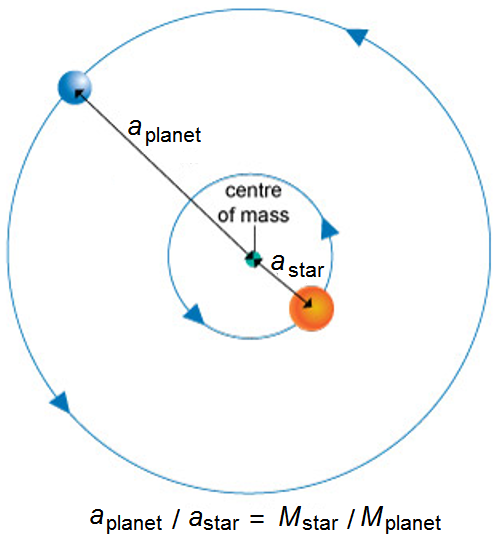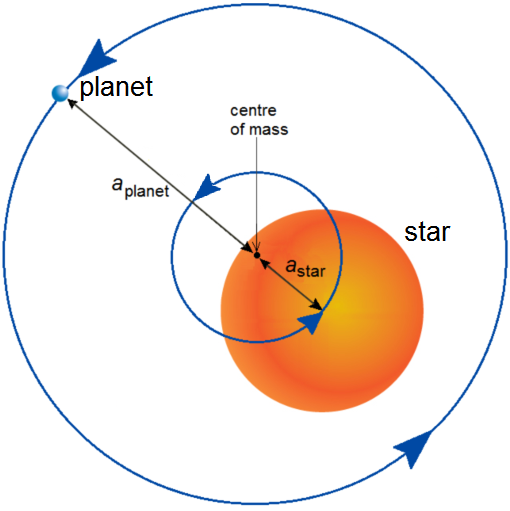Science, Maths & Technology

### Become an OU studentAn introduction to exoplanets

Start this free course now. Just create an account and sign in. Enrol and complete the course for a free statement of participation or digital badge if available.

# 3.1  Gravity, orbits and see-saws

The clever technique used to find 51 Peg b relies on gravity. Gravity is the attraction of anything that has mass to everything else that has mass. For objects in everyday life this effect is too small to be noticeable. For objects as big as planets and stars, however, gravity makes a big difference.

Gravity holds us to the surface of the Earth, and gravity keeps the Earth in orbit around the Sun. In the same way, gravity keeps 51 Peg b in orbit around 51 Peg. But the important thing about gravity is that the force between any two objects is equal and opposite.

That means that 51 Peg b is pulling its star towards it with exactly the same force as the star is pulling on 51 Peg b. So why does 51 Peg b orbit the star, and not the other way around?

Actually, 51 Peg b doesn’t orbit the star. The star and its planet are both in orbit. They both orbit a point in space called the common centre of mass. The word ‘common’ indicates that it is shared, in common, between the star and the planet. This is true for our own Solar System too, where there is more than one planet to consider: all of the masses in the Solar System, including the Sun, orbit their mutual centre of mass. Because the Sun has most of the mass in the Solar System, people often say that everything else in the Solar System orbits the Sun. This is almost, but not exactly, correct.

Scientists can work out the exact position of each large mass in the Solar System, as was demonstrated by the thrilling landing of the Rosetta space probe’s Philae lander on the surface of a comet. To do this requires more calculations than you have time for in this course, but you will cover the underlying principles. For the purposes of this course you might be relieved to know that you will stick to the simpler case of a single planet and its star in orbit.

Figure 2 shows how a planet and star orbit their common centre of mass. As you’d expect, the common centre of mass is always closer to the more massive object, the star. In fact, there is a more mathematical relationship between the masses of the orbiting bodies and their distance to the centre of mass. Dividing the mass of the star by the mass of the planet gives the same answer as dividing the distance of the planet from the common centre of mass by the distance of the star from the common centre of mass. This fact is shown in the diagram: the ratio of the masses (Mstar /Mplanet) is equal to the ratio of the distances from the common centre of mass (aplanet /astar). So, the larger Mstar is, the smaller astar is, as expected.Figure 2  Orbit of a star and planet around their common centre of mass. M represents the mass and a represents the distance from the centre of mass (the symbol a is conventionally used by astronomers rather than the d that you might expect).

Because the mass of the star, Mstar, is so much larger than the mass of the planet, Mplanet, the common centre of mass is often inside the star (Figure 3). But, crucially, the common centre of mass is not at the centre of the star. This means that the star wobbles a bit as the planet goes around it.Figure 3  Orbit of a star and planet around their common centre of mass where the mass of the star is much greater than the mass of the planet

This video [Tip: hold Ctrl and click a link to open it in a new tab. (Hide tip)] animates the orbits of a star and planet where the common centre of mass lies inside the star (marked with a small cross) similar to the situation illustrated in Figure 3.

You can think of this like two people on a see-saw – say a small child and her father. To make sure that the see-saw is perfectly balanced, the heavier father will have to sit close to the pivot, while the little girl sits right at the end of the seat. As the see-saw bounces, the father barely moves at all but the little girl moves a lot.

This same thing happens with the planet and its star. The planet moves in a wide circle around the common centre of mass, while the star moves in a much tighter one. Because stars are so much bigger than planets, the situation in fact resembles a sumo wrestler and a hamster on a see-saw! Sumo wrestlers are about 3000 times the mass of a dwarf hamster, just as the Sun is about 3000 times the mass of Saturn. If you can imagine this, the sumo wrestler would have to literally sit on top of the see-saw’s pivot. In a similar way, the common centre of mass is inside the star.